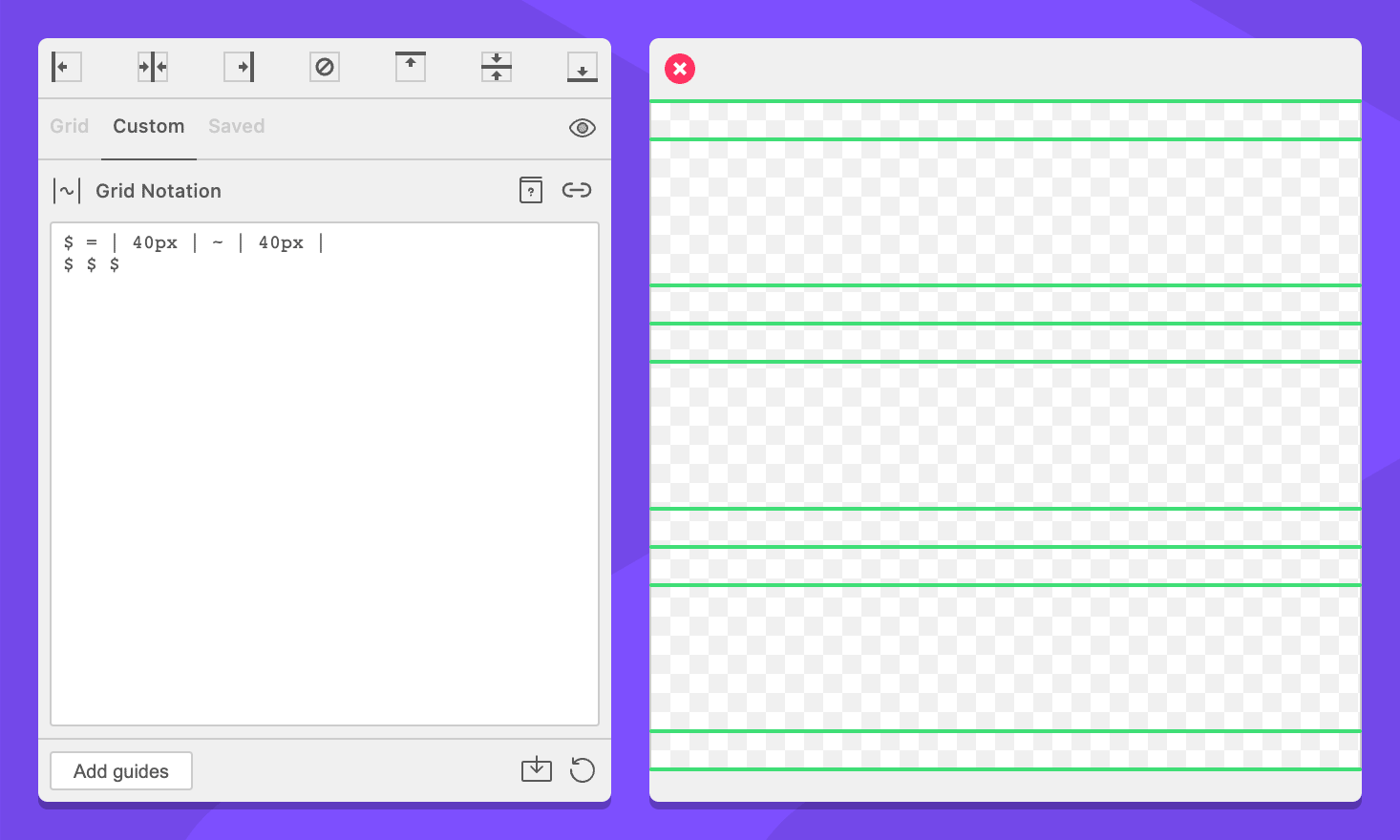# Variables

Variables enable you to define and reuse collections of commands within a grid. Variables need two parts: a declaration and a reference.

## Variable declaration

Format: `\$<ID> = <commands>`
In words: “This variable equals these commands”

``````# Examples:
\$ = | 10px |
\$myGrid = | 1in | .25in |``````

When you declare a variable, you tell GuideGuide to remember a group of commands for later use. You write a variable with the dollar sign `\$` followed by an optional string of letters and numbers.

Declaring a variable does not do anything, it just saves the commands for later use. To use the variable, you must reference it.

Variables can be complete grids, and support all commands, as well as options.

## Variable references

Format: `\$<id>`
In words: “Replace this variable with the commands it represents”

``````# Examples:
| \$ |
| 10px | \$A | 10px |``````

Referencing a variable tells GuideGuide to replace it with the commands that have been declared for that variable. You cannot reference a variable before it is declared. Doing so will cause an Undefined Variable error.

For example, a three column Grid using multiples of a variable command.

``````\$ = | 40px | ~ | 40px |
\$ \$ \$``````The first line declares the variable and the second line references it three times. GuideGuide sees it like this:

``| 40px | ~ | 40px | 40px | ~ | 40px | 40px | ~ | 40px |``

Variables may any command. However, there are some limitations when repeating commands.

## Built in variables

GuideGuide comes with two built in variables, `\$WIDTH` and `\$HEIGHT` which can be used to reference the size of the context.

These variables are helpful to place guides in one axis relative to calculations from the other axis.

``````# This will create a square that is as tall
# as the width of the available space
| \$WIDTH | (v)
| \$WIDTH | (h)``````## Variable flattening

When a variable is referenced, grid notation replaces the variable reference with the commands from that variable.

``````\$1 = 10px

\$2 = \$1
# Becomes: \$2 = 10px

| \$2 |
# Becomes: | 10px |``````

Some commands, such as percentage units and wildcards have to be calculated. These commands are calculated as soon as there is enough information to do so.

This concept is best explained through examples. In all scenarios below, the grid context has a size of 100px.

``````\$1 = ~

\$2 = \$1
# Becomes: \$2 = ~

\$3 = \$1 | \$2
# Becomes: ~ | ~

| \$3 |
# Becomes: | 50px | 50px |``````

Inside the variables, the wildcard stays a wildcard because the variables do not have an explicit size. However, when the variable is referenced in a grid where the implicit grid size is available, the wildcard gets calculated.

Now, consider a scenario where we set a size on one of the variables

``````\$1 = ~

\$2 = \$1 (75px)
# Becomes: \$2 = 75px

\$3 = \$1 | \$2
# Becomes: ~ | 75px

| \$3 |
# Becomes: | 25px | 75px |``````

Let’s walk through that line by line.

``\$1 = ~``

First, we create a variable that holds a wildcard.

``````\$2 = \$1 (75px)
# Becomes: \$2 = 75px``````

We define a new variable that references our wildcard variable. We also define the size of the grid as 75px. Because grid notation has the size information, the wildcard reference in `\$2` gets calculated.

``````\$3 = \$1 | \$2
# Becomes: ~ | 75px``````

The `\$3` variable doesn’t include a size, so the wildcard in `\$1` gets flattened as a wildcard. The wildcard in `\$2` was already calculated, so it is flattened as its calculated value.

``````| \$3 |
# Becomes: | 25px | 75px |``````

In the final step, we have the implicit size of the grid context which is 100px so grid notation calculates the final wildcard.

These mechanics become relevant when using blocks as they allow us reference calculated values between grids.